# Mathematics - Signals And Systems - System Classification with Examples[Image1]

## Introduction

Hey it's a me again @drifter1!

Today we continue with my mathematics series about Signals and Systems in order to cover System Classification, again with Full-On Examples.

So, without further ado, let's get straight into it!

## System Classification

Systems take in signals as an input and output new signals. The inputs are also called excitation, whilst the output is the system's response. Depending on how the input "affects" their output, these systems can be classified into specific categories:

• Linear and Non-Linear Systems → Linearity property
• Time Variant and Time Invariant Systems → Time Variance/Invariance property
• Linear Time Variant (LTV) and Linear Time Invariant (LTI) Systems → we will mostly cover LTI Systems
• Static and Dynamic Systems → Memory property
• Causal and Non-Causal Systems → Causality property
• Invertible and Non-Invertible Systems → Invertibility property
• Stable and Unstable Systems → Stability property
Let's explain each category/classification and get into full-on examples to help explain them better.

## Linear and Non-Linear Systems

A system T is considered linear when it satisfies the superposition principle, which is basically two properties:• Homogeneity:Combining these two properties, a system satisfies Linearity when: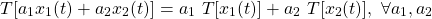### Example 1

Consider the system: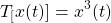Let's find out if the system is linear or non-linear.

For the signals x1(t) and x2(t):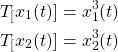Let's now check if the Linearity conditions are met, starting off with Additivity.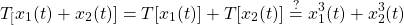The system's response is:After applying a Cube Formula/Identity the system's response ends up being different then expected by the Additivity property. This means that the system T doesn't satisfy Linearity. Thus, we classify the system as non-linear.

### Example 2

An example of a linear system is: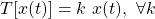Because:and so the linearity property is satisfied!

## Time Variant and Time Invariant Systems

A system is satisfiying time-variance when its input and output vary with time. Else, the system is considered time-invariant.

More specifically a system is time-invariant when:This basically means that the relationship between the input and output is time-independent.

On the other hand, a system is time-variant when: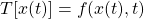In this case, there is an explicit time-dependence between the input and output.

### Example

Consider the following system:Let's find out if the system is time-variant or invariant.

For a random input x1(t), the system response is: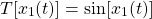Let's time-shift the signal x1(t) in order to get a new signal x2(t):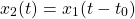For this signal, the system response is: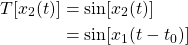Similarly, for the signal x1(t - t0) the system response is: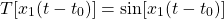Thus, the response of the system for x2(t) and x1(t - t0) is the same, meaning that the system is time-independent.

## Linear Time Variant (LTV) and Linear Time Invariant (LTI) Systems

• A system is classified as LTV when it satisfies both Linearity and Time Variance
• A system is classified as LTI when is satisfies both Linearity and Time Invariance
I will not get into examples as we already covered each property extensively. LTI systems will be covered thoroughly in this series of articles...

## Static and Dynamic Systems

Static systems are memory-less systems, meaning that the output depends only on the present/current input. Contrarily, dynamic systems are memory systems. So, the output depends upon the present and previous inputs.

### Example 1

Consider the system: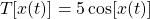The system response for present time t0 = 0 is: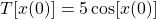Therefore, the system is classified as static or memory-less, as the response depends only on the present input x(t0) = x(0).

### Example 2

Let's now consider the system:The system response for present time t0 = 0 is:
Thus, because the output depends on previous input, x(t-1), the system is classified as dynamic. We could also say that this system satisfies the memory property.

## Causal and Non-Causal Systems

Systems whose response depends upon present and past input but not future input are considered causal, whereas systems that depend on past, present and future input are considered non-causal.

### Example

All examples up to this point where Causal systems, as the system response was only dependent of present and past input. So, let's get into an example of a non-causal system.

Consider the following system: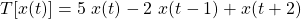For present time t0 = 0 the system resposne is:meaning that the output depends upon the present input x(0), past input x(-1) and the future input x(2).

This system is classified as non-causal.

## Invertible and Non-Invertible Systems

A system is considered invertible if the input of the system can be recovered from the system's output. Similarly, a system is non-invertible when there is no way to recover the input from the output.

So, if y(t) is the response of the system T for input x(t), then their exists another system T -1 with output x(t) for input y(t). The system T -1 is the "inverted" system of T.

Mathematically speaking:### Example 1

Consider the system:For any input x2(t) = - x1(t) we have:Because two different inputs lead to the same output the system is non-invertible.

### Example 2

Let's now consider the system: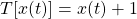Its easy to notice that any input x(t) leads to different outputs, meaning that the system is invertible. In general, any invertible function can be used to "construct" invertible systems.

## Stable and Unstable Systems

Last but not least, systems are considered stable when the output is bounded for bounded input. In opposition, systems are considered unstable when the output is unbounded for bounded input.

`Note that a bounded signal has finite amplitude.`

### Example 1

Consider the system:Using the unit step function u(t) as the input, the output of the system is: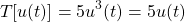And so the system can be classified as stable.

### Example 2

Let's lastly also consider the system: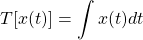Using u(t) as the input, the output is the ramp signal, r(t):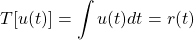which is an unbounded signal, because the amplitude goes to infinity when t → ∞.

So, this final system can be classified as unstable.

## RESOURCES:

### Images

1.## Final words | Next up

And this is actually it for today's post! Till next time!

See Ya!Keep on drifting!

H2
H3
H4
3 columns
2 columns
1 column